##Maclaurin's conic generation method

Consider all triangles ABC whose sides {AB, BC, CA} pass correspondingly through three fixed points {C*,A*,B*} and two of its vertices {B,C} lie correspondingly on two fixed lines {A1X,A1Y}. Then its vertex A describes a conic passing through points {A1, B*, C*}. The conic passes also through the intersection points {B1,C1} of line-pairs (A1X,A*B*) and (A1Y,A*C*).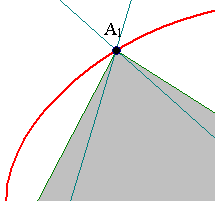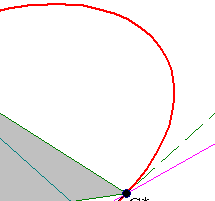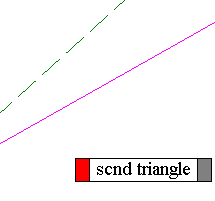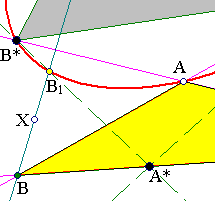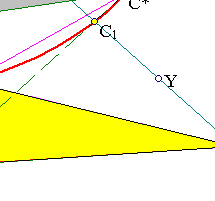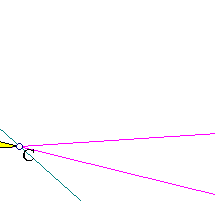The proof is modeled as an exercise in projective coordinates with respect to the fixed points {A*,B*,C*} and with coordinator the fixed point A1. In this system A1=A*+B*+C*. Line BC passing through A* has the form:
y-kz=0 (with variable k),
and lines {A1X, A1Y} correspondingly
x-ay-bz=0,
x-a'y-b'z=0. with fixed (a,b), (a',b') satisfying 1=a+b=a'+b' (*), since A1 is on these lines.
The coordinates of {B,C} are correspondingly:
(ka+b, k, 1) and (ka'+b', k, 1).
Then lines {BC*,CB*} obtain the form:
-kx+(ka+b)y=0 and -x+(ka'+b')z=0.
And eliminating k from them leads to equation:
ba'yz = (b'z-x)(ay-x) (**)
representing a conic passing through B*(0,1,0), C*(0,0,1) and A1(1,1,1) (use (*)) as stated. The two additional points are the intersection points of line-pairs (x-ay-bz=0, z=0) and (x-a'y-b'z=0, y=0) which are easily calculated to be (a,1,0) and (b',0,1) and satisfy equation (**).

##Inverse theorem

The theorem admits an inverse in the obvious form: Given a conic (c) and three points {A1,B*,C*} on it, consider two fixed lines {A1X, A1Y}. For every point A of the conic define the intersection points {B,C} of line-pairs (AC*,A1X) and (AB*,A1Y). Then line BC, as A varies on the conic, pivots around point A*, which is the intersection point of line-pairs (B*B1,C*C1), where {B1,C1} are the intersection points of the conic with lines {A1X,A1Y}.

To prove the inverse one can use Maclaurin's construction to define a conic (c') with the given data. This conic then will have with (c) the common points {A1,B*,C*,B1,C1}, thus the two conics will coincide (see also Remark-2 below).

Remark-1 Maclaurin's construction is a special case of the Chasles-Steiner (should his name be mentioned in this context since he is older than the other two) way of generating conics through intersections of homologous lines of two pencils which are in homographic correspondence. Here the two pencils are those of lines passing through B* and C*. The line pivoting at A* defines the homographic correspondence of the two pencils. To line B*C it corresponds line C*B. The homography is recognized also in the preceding calculation. In fact, B can be writen B=k(a,1,0)+(b,0,1)=kB'+B'', the two points B',B'' lying on line A1X. Analogously C=k'(a',1,0)+(b',0,1)=k'C'+C'', the two points C',C'' lying on line A1Y. The homographic correspondence is represented through equation k'=k. In this relation k, k' are considered as projective coordinates of the pencils of lines at C* and B* correspondingly.

Remark-2 Of particular interest is the case in which lines {A1X, A1Y} coincide with sides {A1B*, A1C*} of triangle A1B*C*. In that case conic (c) becomes tangent to lines {A*B*,A*C*} at corresponding points {B*,C*}. A* is the pole of line B*C* (see figure below). The inverse of Maclaurin's construction becomes Pascal's theorem for quadrilaterals: For quadrilateral A1B*AC*, the intersection points {B,C} of pairs of opposite sides and the tangents at pairs of opposite vertices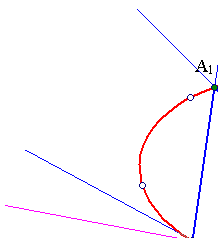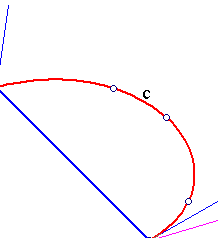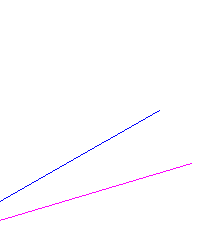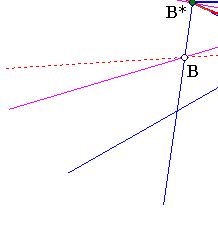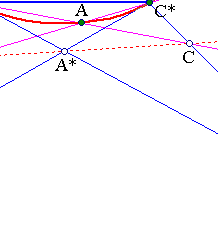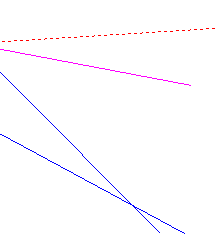meet on a line (BC). Maclaurin's construction in this case shows that the quadrilaterals A1B*AC* created by inverting Pascal's theorem (for quadrilaterals and for {B,C} on lines {A1B*,A1C*} and collinear with A*) are all inscribed in the same conic.

Remark-3 Analogous remark applies to the general case, in which A1B*B1AC1C* is a hexagon inscribed in the conic (c). By Pascal's theorem opposite sides intersect along a line and this could give a proof of the inverse of Maclaurin's construction. Maclaurin's construction itself is a variation on the inverse of Pascal's theorem, showing that when {B,A*,C} are collinear then the respective hexagon is on a conic (c) and even (c) is the same by various choices of {B,C}.

Remark-4 Given five points like {A1,B*,B1,C*,C1} Maclaurin's construction gives a means to construct arbitrary many additional points of the conic passing through those five points. The additional points result as vertices of triangles ABC pivoting around A* (A* being also determined through the five given points).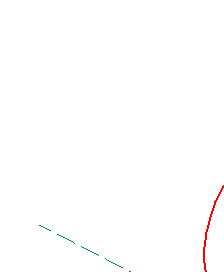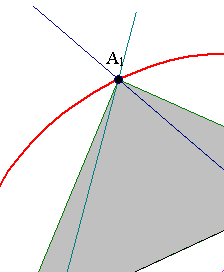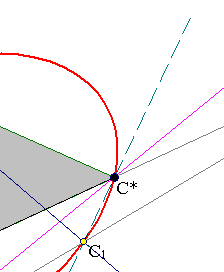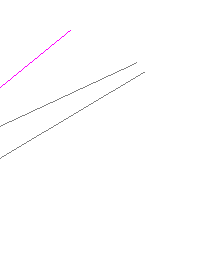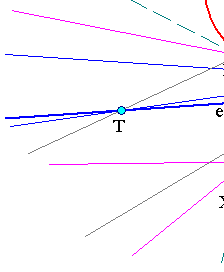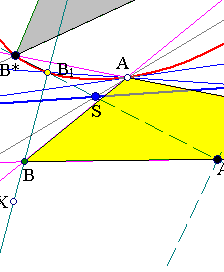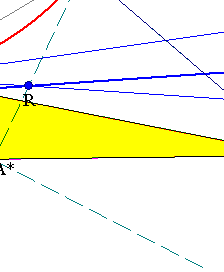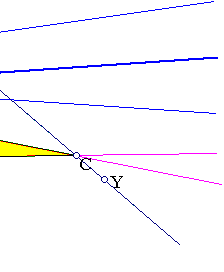Remark-5 The tangent at A can be easily located by means of the theorem of Pascal applied to pentagon B*B1AC1C*. By this theorem the intersection points {T,R,S} of the tangent and line B*C* as well as of the line-pairs (B1A,C1C*) and (B*B1, C1A) correspondingly will lie on a line (e). Thus the tangent AT can be constructed by first finding {S,R}, then T as intersection of line-pairs (SR,B*C*).

### See Also

AntiparallelHyperbola.html
ConicsMaclaurin.html
ConicsMaclaurin2.html

### References

Baker, H. F. Plane Geometry New York, Chelsea Publishing Company 1971, p. 77.
Salmon, G. A treatise on conic sections London 1855, Longmans, p. 230

 Produced with EucliDraw© http://users.math.uoc.gr/~pamfilos/